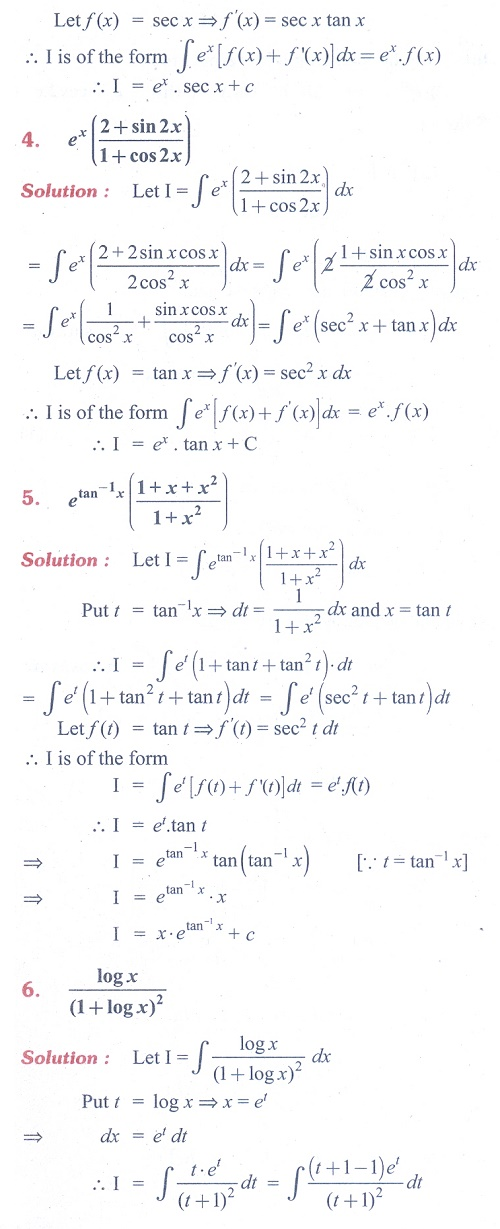Home | | Maths 11th std | Exercise 11.9: Integrals of the form e pow(ax) cos bx and e pow(ax) sin bx

# Exercise 11.9: Integrals of the form e pow(ax) cos bx and e pow(ax) sin bx

Maths Book back answers and solution for Exercise questions - Mathematics : Integral Calculus - Integrals of the form e pow(ax) cos bx and e pow(ax) sin bxTags : Problem Questions with Answer, Solution | Mathematics , 11th Mathematics : UNIT 11 : Integral Calculus
Study Material, Lecturing Notes, Assignment, Reference, Wiki description explanation, brief detail
11th Mathematics : UNIT 11 : Integral Calculus : Exercise 11.9: Integrals of the form e pow(ax) cos bx and e pow(ax) sin bx | Problem Questions with Answer, Solution | Mathematics